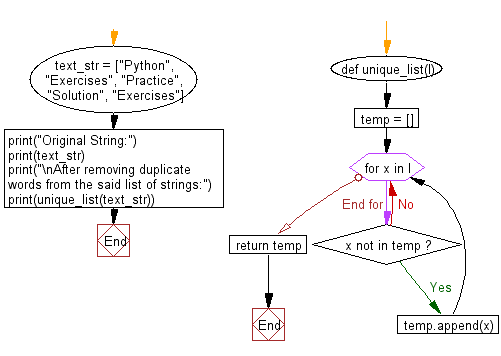﻿ Python: Remove duplicate words from a given list of strings - w3resource# Python: Remove duplicate words from a given list of strings

## Python List: Exercise - 136 with Solution

Write a Python program to remove duplicate words from a given list of strings.

Sample Solution:

Python Code:

``````def unique_list(l):
temp = []
for x in l:
if x not in temp:
temp.append(x)
return temp

text_str = ["Python", "Exercises", "Practice", "Solution", "Exercises"]
print("Original String:")
print(text_str)
print("\nAfter removing duplicate words from the said list of strings:")
print(unique_list(text_str))
```
```

Sample Output:

```Original String:
['Python', 'Exercises', 'Practice', 'Solution', 'Exercises']

After removing duplicate words from the said list of strings:
['Python', 'Exercises', 'Practice', 'Solution']
```

Flowchart:## Visualize Python code execution:

The following tool visualize what the computer is doing step-by-step as it executes the said program:

Python Code Editor:

Have another way to solve this solution? Contribute your code (and comments) through Disqus.

What is the difficulty level of this exercise?

Test your Python skills with w3resource's quiz

﻿

## Python: Tips of the Day

Floor Division:

When we speak of division we normally mean (/) float division operator, this will give a precise result in float format with decimals.

For a rounded integer result there is (//) floor division operator in Python. Floor division will only give integer results that are round numbers.

```print(1000 // 300)
print(1000 / 300)```

Output:

```3
3.3333333333333335```